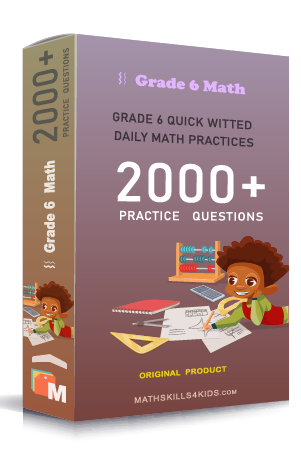# Grade 6 Operations with integers worksheets with solutions

Subject
Math

Resource Type
Worksheets, Printables, Homeschool

Format
PDF (14.5 MB | 22 pages → Exercises + Solutions)

• ### -15% OFF Over \$100

Promo Code: TOPLA

After Purchase

\$3.15

This package allows you to practice the following skills

Finding the sum of integers

Subtracting integers using counters

Subtracting integers

Adding and subtracting integers: find the sign

Adding and subtracting integers: input/output tables

Multiplying integers: find the sign

Finding the products of intergers

Dividing integers: find the sign

Finding the quotient of integers

This product is a part of the Mega Pack
* Grade 6 quick-witted daily math practices *